# Imperial and Metric Units' Online Conversion

Standard  |  Mobile  |  Articles  |  Domain name checker

Area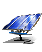Energy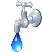Flowrate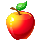Force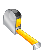Length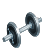Mass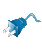Power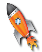Pressure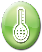Temperature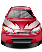Velocity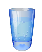Volume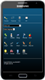App

 Select a unit to convert Acres Ancient Greek Units Ancient Roman Units Apothecaries' System Ares BarsBarrels per day Baryes BTUs BTUs per hour (BTU/h) BTUs per minute (BTU/min) BTUs per second (BTU/s) Degrees Celsius (°C) Centimeters (cm) Centimeters per second (cm/sec) Chains Crinals Cubic centimeters (cc, cm3, cu cm) Cubic centimeters per second (cc/s, cm3/s, cu cm/s) Cubic centimeters-atmosphere (cc-atm) Cubic decimeters (cu dm, dm3) Cubic feet (cu ft, ft3) Cubic feet per minute (cu ft/min, ft3/min) Cubic feet per second (cu ft/s, ft3/s) Cubic inches (cu in, in3) Cubic inches per minute (cu in/min, in3/min) Cubic inches per second (cu in/s, in3/s) Cubic meters (m3) Cubic meters per hour (m3/h) Cubic yards (cu yd, yd3) Cubic yards per minute (cu yd/min, yd3/min) Decimeters (dm) Drams Dynes Ergs per second (ergs/s) Degrees Fahrenheit (°F) Fluid drachmas Fluid ounces (fl oz) Feet (ft) Feet per hour (ft/h) Feet per minute (ft/min) Feet per second (ft/s) Foot-pound-forces (ft-lbf) Foot-pound-forces per hour (ft-lbf/h) Foot-pound-forces per minute (ft-lbf/min) Foot-pound-forces per second (ft-lbf/s) Furlongs Gallons (gal) Gallons per day (gal/d) Gallons per minute (gal/min) Gallons per second (gal/s) Gigawatts (GW) Gills Grains Grams (g) Gram-calories (g-cal) Hectares (ha) Hectometers (hm) Hundredweights (cwt) Inches (in) Inches H2O at 15.56 degrees Celsius Inches Hg at 15.56 degrees Celsius Inch-pound-forces (in-lbf) IST calories Joules (J) Joules per second (J/sec) Kelvin (K) Kilocalories (kcal) Kilograms (kg) Kilogram-meters per square second (kg m/s2) Kilogram-calories (kg-cal, kgcal, kg cal) Kilogram-forces (kgf, kg f, kg-f) Kilogram-forces per square centimeter (kgf/cm2, kg-f/cm2) Kilogram-forces per square meter (kgf/m2, kg-f/m2) Kilometers (km) Kilometers per hour (km/h, km/hour) KiloPascal(kPa) Kilowatts (kW) Kilowatts per hour (kWh) Kips Knots (kn) League Liters (l) Liters per minute (l/min) Liters per second (l/s) Long tons (imperial tons) MegaPascal(mPa) Megawatts (MW) Meters (m) Meters per minute (m/min) Meters per second (m/s) Metric horsepower (metric HP) Miles Miles per hour (mph, miles/h, mi/h) Miles per minute (mi/min, miles/min) Milligrams (mg) Milliliters (ml) Millimeters (mm) Miner inches Newton (N) Ounces (oz) Ounce-forces (oz-f) Pascal(Pa) Pints Pounds (lb) Pounds per square foot (lb/ft2, lb/sq ft) Pounds per square inch (lb/in2, lb/sq in) Poundals Poundals per square foot Poundals per square inch Pounds-CHU Pound-forces (lbf, lb-f) Quarts Quarters Rods Short tons per square foot Slugs Square centimeters (sq cm, cm2) Square decimeters (sq dm, dm2) Square feet (sq ft, ft2) Square kilometers (sq km, km2) Square meters (sq m, m2) Square miles (sq mi, sq miles, mi2) Square millimeters (sq mm, mm2) Square yards (sq yd, yd2) Stones Thermocalories Tons Tons (refrigeration) Tonnes (metric tons) Torrs U.S. gallons (US gal) U.S. horsepower per hour (US HP/h) U.S. pints U.S. tons Watts (W) Watts per hour (Wh, W-h, W h, W/h) Yards (yd) ________________ Other units Cooking Measure Units Currency Units Internet Reach Stats

 Masston hundredweight (cwt) pound US ton quarter stone ounce dram grain tonne kilogram gram milligram In ton hundredweight (cwt) pound US ton quarter stone ounce dram grain tonne kilogram gram milligram
 Lengthmile furlong chain rod yard foot inch kilometer hectometer meter decimeter centimeter millimeter In mile furlong chain rod yard foot inch kilometer hectometer meter decimeter centimeter millimeter
 Volumegallon quart pint gill fluid ounce fluid drachm US gallon US pint cubic yard cubic foot cubic inch cubic meter liter cubic decimeter cubic centimeter milliliter In gallon quart pint gill fluid ounce fluid drachm US gallon US pint cubic yard cubic foot cubic inch cubic meter liter cubic decimeter cubic centimeter milliliter
 Area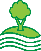square mile acre square yard square foot square kilometer hectare are square meter square decimeter square centimeter square millimeter In square mile acre square yard square foot square kilometer hectare are square meter square decimeter square centimeter square millimeter
 Velocitymile per hour centimeter per second foot per hour foot per minute foot per second kilometer per hour knot meter per minute meter per second mile per minute In mile per hour centimeter per second foot per hour foot per minute foot per second kilometer per hour knot meter per minute meter per second mile per minute
 EnergyBritish Thermal Unit (BTU) calorie (IST) calorie (thermo) cubic centimeter-atmosphere foot-pound-force gram-calorie US horsepower per hour inch-pound-force kilogram-calorie kilowatt per hour pound-CHU watt per hour joule kilocalorie In British Thermal Unit (BTU) calorie (IST) calorie (thermo) cubic centimeter-atmosphere foot-pound-force gram-calorie US horsepower per hour inch-pound-force kilogram-calorie kilowatt per hour pound-CHU watt per hour joule kilocalorie
 ForceNewton crinal dyne grain kilogram-force kilogram-meter per square second kip ounce-force pound-force poundal slug imperial ton (long ton) In Newton crinal dyne grain kilogram-force kilogram-meter per square second kip ounce-force pound-force poundal slug imperial ton (long ton)
 Flowrategallon per minute barrel per day cubic centimeter per second cubic inch per second cubic inch per minute cubic foot per minute cubic foot per second cubic meter per hour cubic yard per minute gallon per day gallon per second liter per minute liter per second miner's inch In gallon per minute barrel per day cubic centimeter per second cubic inch per second cubic inch per minute cubic foot per minute cubic foot per second cubic meter per hour cubic yard per minute gallon per day gallon per second liter per minute liter per second miner's inch
 Powerwatt BTU per hour BTU per minute BTU per second foot-pound-force per hour foot-pound-force per minute foot-pound-force per second gigawatt joule per second metric horsepower kilowatt megawatt ton (refrigeration) erg per second In watt BTU per hour BTU per minute BTU per second foot-pound-force per hour foot-pound-force per minute foot-pound-force per second gigawatt joule per second metric horsepower kilowatt megawatt ton (refrigeration) erg per second
 PressurePascal bar barye inch H2O (15.56 °C) inch Hg (15.56 °C) kilogram-force per square centimeter kilogram-force per square meter kiloPascal megaPascal pound per square foot pound per square inch poundal per square inch poundal per square foot short ton per square foot torr In Pascal bar barye inch H2O (15.56 °C) inch Hg (15.56 °C) kilogram-force per square centimeter kilogram-force per square meter kiloPascal megaPascal pound per square foot pound per square inch poundal per square inch poundal per square foot short ton per square foot torr
 Temperature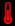Select  Conversion  Celsius  -->  Fahrenheit   Celsius  -->  Kelvin  Fahrenheit  -->  Celsius  Fahrenheit  -->  Kelvin  Kelvin  -->  Fahrenheit  Kelvin  -->  Celsius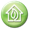#### Worldwide use of the Metric System and Date of Metrication

* The United States of America, Burma and Liberia have not yet adopted the Metric System (SI).##### Conversion Table

 U.S. unit Metric equivalent Metric unit U.S. equivalent
 Inch (in) Centimeter (cm)
 2.54 centimeters 0.394 inch

 Foot (ft) Meter (m)
 0.305 meter 3.28 feet

 Yard (yd) Meter (m)
 0.914 meter 1.09 yard

 Mile (mi) Kilometer (km)
 1.61 kilometer 0.621 mile

 Square inch (in2) Square centimeter (cm2)
 6.45 square centimeters (cm2) 0.155 square inch (in2)

 Square foot (ft2) Square meter (m2)
 0.093 square meter (m2) 10.8 square feet (ft2)

 Square yard (yd2) Square meter (m2)
 0.836 square meter (m2) 1.2 square yard (yd2)

 Square mile (mi2) Square kilometer (km2)
 2.56 square kilometers (km2) 0.386 square mile (mi2)

 Acre Hectare (ha)
 0.405 hectare 2.47 acres

 Cubic foot (ft3) Cubic meter (m3)
 0.028 cubic meter (m3) 35.28 cubic feet (ft3)

 Quart (qt) Liter (l)
 0.946 liter 1.06 quart

 Gallon Cubic inch (in3)
 231 cubic inches (in3) 0.0043 gallon

 Ounce (oz) Gram (g)
 28.3 grams 0.035 ounce

 Pound (lb) Kilogram (kg)
 0.454 kilogram 2.2 pounds

###### Units per conversion type
Area
 square meter (m2) are (a) = 100 sq. meters (m2) hectare (ha) = 100 Ares = 10 000 sq. meters square kilometer (km2) = 100 hectares = 10000 Ares = 1 000 000 sq. meters square mega meter (Mm2) = 1 000 000 000 000 sq. meters square foot = 144 sq. inches = 0.09290304 sq. meters square yard = 9 sq. feet = 0.83612736 sq. meters square perch = 30.25 sq. yards = 25.2928526 sq. meters acre = 10 sq. chains = one furlong by one chain = 160 sq. perches = 4840 sq. yards = 43 560 sq. feet = 4046.8564224 sq. meters square mile = 640 acres = 2.589988110336 sq. kilometers

Metric System Imperial
1 square centimeter [cm2] 100 square millimeters 0.001076391 square feet
1 square meter [m2] 10000 square centimeters 1.19599004630 square yard
1 hectare [ha] 10000 square meters 2.47105381467 acres
1 square kilometer [km2] 100 hectares 0.38610215854 square mile

Imperial Metric System
1 square inch [in2] 6.4516 square centimeters
1 square foot [ft2] 144 square inches 0.09290303999 square meter
1 square yard [yd2] 9 square feet 0.83612736 square meter
1 acre 4840 square yards 4046.8564224 square meters
1 square mile 640 acres 2.589988110336 square kilometers

Length

 Main length units: meter centimeter kilometer inch foot yard mile light-year (astronomical unit) parsec (astronomical unit) angstrom (microscopic unit)

Metric System Imperial
1 millimeter [mm] 0.03937007874 inch
1 centimeter [cm] 10 millimeters 0.3937007874 inch
1 meter [m] 100 centimeters 1.09361329833 yard
1 kilometer [km] 1000 meters 0.62137119223 mile

Imperial Metric System
1 inch [in] 2.54 centimeters
1 foot [ft] 12 inches 0.3048 meter
1 yard [yd] 3 feet 0.9144 meter
1 mile 1760 yards 1.609344 kilometer

Weight and Mass

 Main weight units: gram (g) = 1⁄1000 kg kilogram (kg) tonne (t)= 1 000 kg electronvolt (eV) atomic mass unit (u) slug (sl) pound (lb) Planck mass (mP) solar mass (M⊙) Compton wavelength Schwarzschild radius

Metric System Imperial
1 milligram [mg] 0.01543209876 grain
1 gram [g] 1000 milligrams 0.03527399072 ounce
1 kilogram [kg] 1000 grams 2.20458553791 pounds
1 tonne [t] 1000 kilograms 0.98418997228 ton

Imperial Metric System
1 ounce [oz] 437.4922839506173 grains 28.3495 grams
1 pound [lb] 16 ounces 0.4536 kilogram
1 stone 14 pounds 6.35 kilograms
1 hundredweight [cwt] 112 pounds 50.8032 kilograms
1 ton 20 hundredweights 1.016064 tonne

Volume

Main volume units:
 cubic inch (cu in) or (in3) cubic foot (cu ft) or (ft3) cubic yard (cu yd) or (yd3) acre foot (acre ft) minim (min) US fluid dram (fl dr) teaspoon (tsp) tablespoon (Tbsp) US fluid ounce (fl oz) jigger (jig) US gill (gi) US cup (cp) US pint (pt) US quart (qt) US gallon (gal) barrel (bbl) hogshead pint (pt) quart (qt) gallon (gal) peck (pk) bushel (bu) fluid ounce (fl oz) gill

Metric System Imperial
1 cubic centimeter [cm3] 0.06102361003 cubic inch
1 cubic decimeter [dm3] 1000 cubic centimeters 0.03531458914 cubic foot
1 cubic meter [m3] 1000 cubic decimeters 1.30794774594 cubic yard
1 liter [l] 1 cubic decimeter 1.75975011548 pint
1 hectoliter [hl] 100 liters 21.9968764435 gallons

Imperial Metric System
1 cubic inch [in3] 16.3871 cubic centimeters
1 cubic foot [ft3] 1728 cubic inch 0.0283169088 cubic meter
1 fluid ounce [fl oz] 28.413125 milliliters
1 pint [pt] 20 fluid ounces 0.5682625 liter
1 gallon [gal] 8 pints 4.5461 liters

Energy

 Main energy units: ergs calories joule kilowatt hour = 3.6 million joules British thermal unit (BTU) = 1055 joules (approx.)

Flow rate

 Definition: volume of fluid passing through a particular surface per unit time (i.e.: cubic meters per minute or cubic feet per minute). Symbol: Q. Also know as: volumetric flow rate volume flow rate rate of fluid flow

Force

 Force units: Newton dyne kilogram-force pound-force poundal

Power

 Main power units: watt (W) = 1 joule per second ergs per second (erg/s) horsepower (hp) = 33 000 foot-pounds per minute = 746 watts metric horsepower [Pferdestärke (PS) or cheval vapeur (CV)] foot-pounds per minute dBm calories per hour kilocalories per hour Btu per hour (Btu/h) tons of refrigeration = 12 000 Btu/h

Pressure

Main pressure units:
 Pascal (Pa) = one Newton per square meter pound per square inch (psi) bar barye (ba) = dyne per square centimeter hectoPascal (hPa) kiloPascal (kPa) millibar (mbar) inch of mercury (inHg) decibar (dbar) = increase of 1 meter (depth) rule of thumb (for scuba divers) standard atmosphere (atm) = 101 325 Pa = 101.325 kPa = 1013.25 hPa millimeters of mercury (mmHg) = one torr inches of mercury millimeter, centimeter, meter, inch and foot of water kip, ton-force (short), ton-force (long), pound-force, ounce-force and poundal per square inch kg-force, or kilopond, per square cm gram-force and tonne-force (metric ton-force per square cm kg-force and tonne-force per square meter sthene per square meter (pieze)

Temperature

 Main temperature units: Kelvin (K) Celsius Fahrenheit electronvolts (eV)= 11 605 K kiloelectronvolts (keV) Rankine

Velocity

 Main velocity units: Meters per second (m s−1 or m/s) Kilometers per hour (km/h) Miles per hour (mph) Knots (nautical miles per hour [kn or kt]) Feet per second (fps or ft/s) Mach number = speed divided by the speed of sound Speed of light in vacuum (c = 299 792 458 m/s)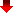About   |   Contact   |   Toolbar   |

Conversion tables and results shown on this website are for information purposes only.  Please contact the webmaster if you find an error on this site.  Although we cannot be held responsible for errors that may have been made, we shall proceed with the correction of any reported error in a timely manner.